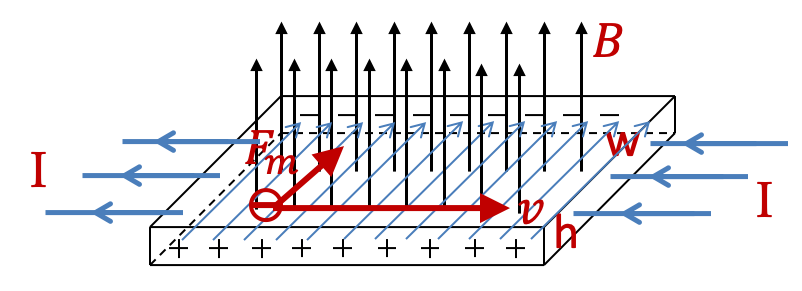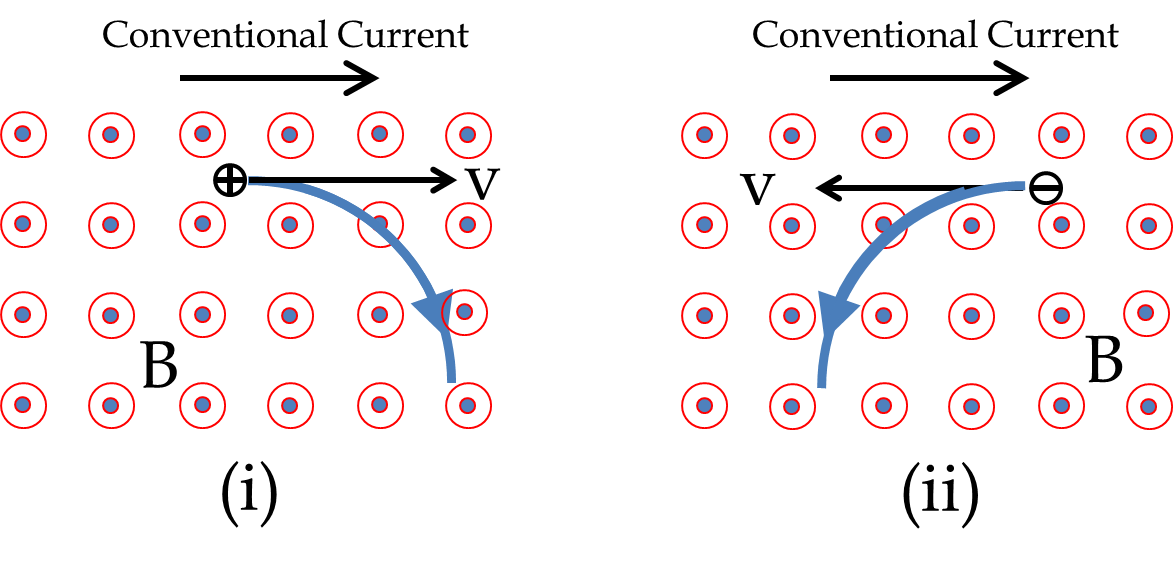## Section38.7The Hall Effect

Hall effect is routinely used to measure magnetic field. Due to the Hall effect, you can obtain a value of magnetic field from easily measurable quantities such as current and voltage.

As a concrete example of Hall effect, let us investigate what happens when a metal plate carrying a steady current is placed in a magnetic field as shown in Figure 38.7.1. You will find that when no current is passing in the metal plate there is no voltage difference between the sides of the plate labeled $+$ and $-$ in the figure but when a current is flowing, a voltage difference develops between the two sides. This voltage is called Hall voltage.Figure 38.7.1. Hall Effect. Voltage across a metal plate develops when steady current flows in the presence of an external magnetic field.

Why does the Hall voltage develop? As shown in Figure 38.7.1, drifting electrons in metal plate experience a magnetic force, which deflect them towards one edge and start to accumulate there. The accumulated charges produce electric field that applies an electric force on the drifting electrons in opposite direction to the magnetic force. When enough charges have moved on the side, electric force balances the magnetic force, similar to what happens in crossed field.

The accumulates charges on the sides means that we will have a potential difference across the plate. This voltage will be denoted by $\Delta \phi_{\perp}\text{,}$ which we will denote below by $V_\perp\text{.}$ We will show that $V_\perp$ is proportional to the magnitude of the magnetic field. Therefore, a Hall probe based on this effect can be constructed to measure magnetic field as we will see below.

The potential difference $V_\perp$ across the plate corresponds to the electric field $\vec E_{\perp}$ in the direction perpendicular to the direction of drift velocity $\vec v\text{.}$ This potential difference is also called Hall voltage, and is often denoted by $V$ rather than $V_\perp$ or $\Delta \phi_\perp\text{.}$

Assuming uniform electric field in the direction perpendicular to the direction of drift velocity $\vec v\text{,}$ we find the following relation between the potential difference and the magnitude of the electric field.

\begin{equation*} V_\perp = E_{\perp}\; w. \end{equation*}

At steady state, electric force perpendicular to the flow balances the magnetic force on each moving electron.

\begin{equation*} \left|-eE_{\perp}\right| = \left|-ev B\right|. \end{equation*}

Replacing $E_{\perp}$ by $V_\perp/w\text{,}$ and solving for $B$ we obtain the following relation.

\begin{equation} B = \frac{V_\perp}{v w}, \label{eq-hall-eff-1}\tag{38.7.1} \end{equation}

which we can write in terms of the current in the plate and the geometric dimensions of the plate as follows.

Let there be $N$ mobile electrons per unit volume and $w$ and $h$ be the width and height of the cross-section area of the plate perpendicular to the current as shown in Figure 38.7.1. Then, steady current $I$ can be shown to be

\begin{equation*} |I| = |-eN v wh|\ \ \Longrightarrow\ \ v = \frac{|I|}{eN wh}. \end{equation*}

Putting this $v$ into Eq. (38.7.1) we obtain magnetic field in terms of two measurable quantities, current through the plate and Hall voltage across the plate.

\begin{equation} B = \frac{V_{\perp}}{|I|}\left( eN wh\right). \label{eq-hall-eff-2}\tag{38.7.2} \end{equation}

This formula based on Hall effect is frequently used for measuring magnetic field by a device called a Hall probe. You first calibrate the instrument for a particular current, and then use formula given in Eq. (38.7.2) to determine an unknown magnitude $B$ from the reading of Hall voltage $V_{\perp}$ by connecting a voltmeter across the plate.

The direction of the magnetic field is obtained by the orientation of the area of the plate. The normal to the plate that gives the maximum reading of the Hall voltage is the direction of the magnetic field.

Nature of Current Carrier by Hall Probe:

Note that if the mobile charges were positively charged rather than electrons, the potential direction will have just the opposite sign. For instance, in some semiconductors the current is actually carried by positively charged entities called holes, where the Hall voltage has just opposite sign of what you would expect if current was due to movement of electrons. In this way, Hall effect also provides information about the nature of charge carriers in a material.

A Hall probe gives a reading of $1.5\ \mu\text{V}$ for a current of $2\text{ A}$ when it is placed in a magnetic field of $1.0 \text{ T}\text{.}$ What is the magnetic field in a region where the reading is $2.0\ \mu\text{V}$ for $1.7 \text{ A}$ of current?

Hint

Use Hall effect formulas

Magnitude $1.57\text{ T}\text{.}$

Solution

Hall probe has the following relation between $B\text{,}$ $\Delta\phi$ and $I\text{.}$

\begin{equation*} B = \alpha\:\dfrac{V_{\perp}}{I}, \end{equation*}

where $\alpha$ is a constant depending on the geometry and electronic property of the probe. We find $\alpha$ from the calibration data, and then find the unknown magnetic field.

\begin{align*} \alpha \amp = B\:\dfrac{I}{V_{\perp}} \\ \amp = 1\:\text{T}\times \dfrac{2\:\text{A}}{1.5\times 10^{-6}\:\text{V}} = 1.33\times 10^6\:\dfrac{\text{TA}}{\text{V}}. \end{align*}

Hence the unknown magnetic field

\begin{align*} B \amp = \alpha\:\dfrac{V_{\perp}}{I} \\ \amp = 1.33\times 10^6\:\dfrac{\text{TA}}{\text{V}} \times \dfrac{2\times 10^{-6}\:\text{V}}{1.7\:\text{A}} = 1.57\:\text{T}. \end{align*}

You know that current in a metal is carried by negatively charged electrons. In semicondutors, the sign of the current carrier may be positive or negative depending on the semiconductor. Hall effect can be used to find the sign.

A rectangular sample of a semiconcting material is placed between the poles of a magnet so that magnetic field is pointed up. When current is passed through the plate in the east direction, the north side of the sample develops a higher potential than the south side. Decide if the current carrier is positively or negatively charged.

Hint

First assume charge carrier is positive and predict what you will see and then see if you need o invert the direction.

Negatively charged.

Solution

We first draw a picture in which we first assume the current is carried by the positive charge as in (i) and predict the end that will become at higher voltage by using the right-hand rule of magnetic force.Fig (i) shows that if the current was due to mobile positive charges to the right, then the bottom part, i.e. South end, will become more positively charged, which will make that end at higher potential compared to the top part.

In Fig. (ii), the north side will be at a higher voltage than the south. Hence the carrier charge in the present problem is negatively charged.

The density carrier is copper is $8.47 \times 10^{28}$ electrons per cubic meter. What will be the Hall voltage reading from a probe made up of $3\ \text{cm} \times 2\ \text{cm} \times 1\ \text{cm}$ $(L\times W\times T)$ copper plate when a current of $1.5\text{ A}$ is passed through it in a magnetic field of $2.5\text{ T}$ perpendicular to the $3\ \text{cm} \times 2\ \text{cm}$ face.

Hint

Use Hall effect.

$4.65\times 10^{-7}\ \text{V}\text{.}$

Solution

Recall the Hall effect formula,

\begin{equation*} B = \dfrac{V_{\perp}}{I}\: n\:e\:w\:h. \end{equation*}

Putting the values from the problem statement, we find the Hall voltage $V_{\perp}\text{.}$

\begin{align*} V_{\perp} \amp = \dfrac{BI}{n\:e\:w\:h} \\ \amp= \dfrac{2.5\: \text{T}\times 1.5\:\text{A}}{8.4\times 10^{28}\:\text{m}^{-3}\times 1.6\times 10^{-19}\:\text{C}\times 0.02\:\text{m}\times 0.03\:\text{m}}\\ \amp = 4.65\times 10^{-7}\:\text{V}. \end{align*}

Hall effect is to be used to find the current carrier density in an unknown material. A Hall voltage $40\ \mu\text{V}$ for $3\text{ A}$ current is observed in a $3\text{ T}$ magnetic field for a rectangular sample with length $2\text{ cm}\text{,}$ width $1.5\text{ cm}$ and height $0.4\text{ cm}\text{.}$ Determine the density of current carriers.

Hint

Use Hall effect formula.

$2.34\times 10^{28}\text{m}^{-3}\text{.}$
Putting the values from the problem statement, we find the Hall voltage $V_{\perp}\text{.}$